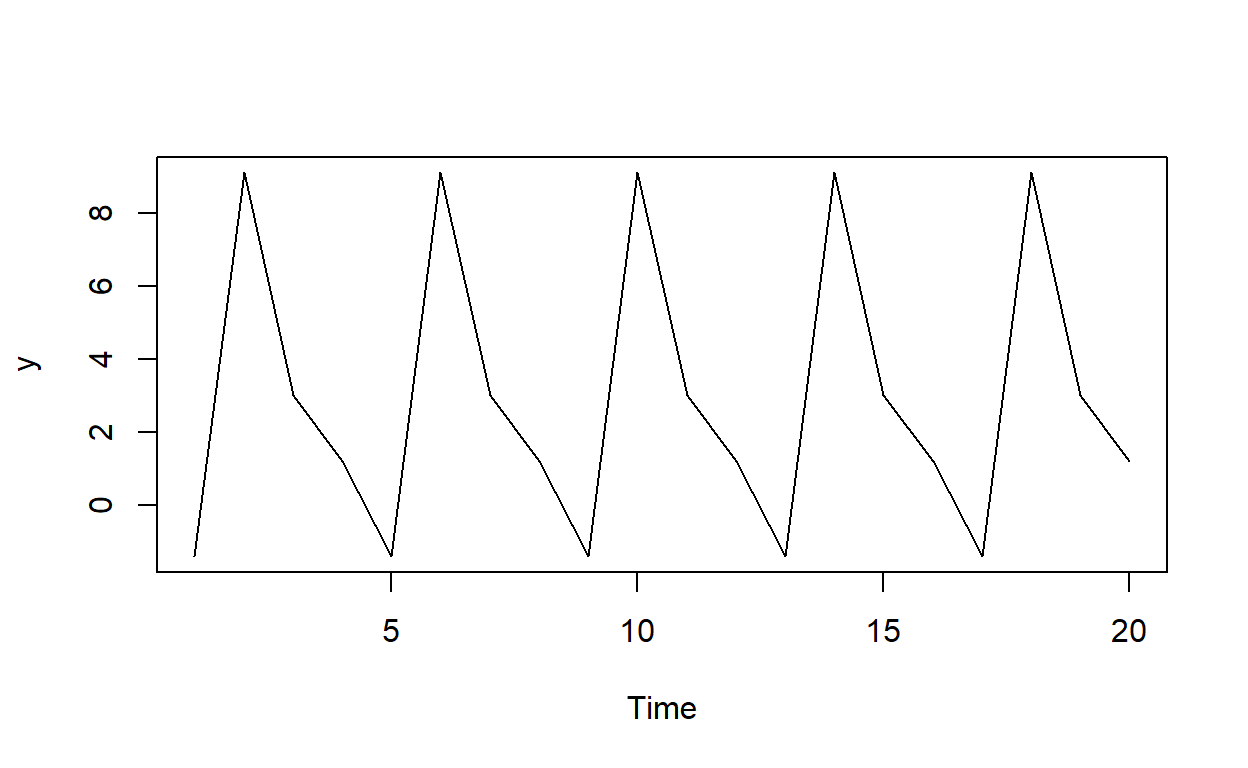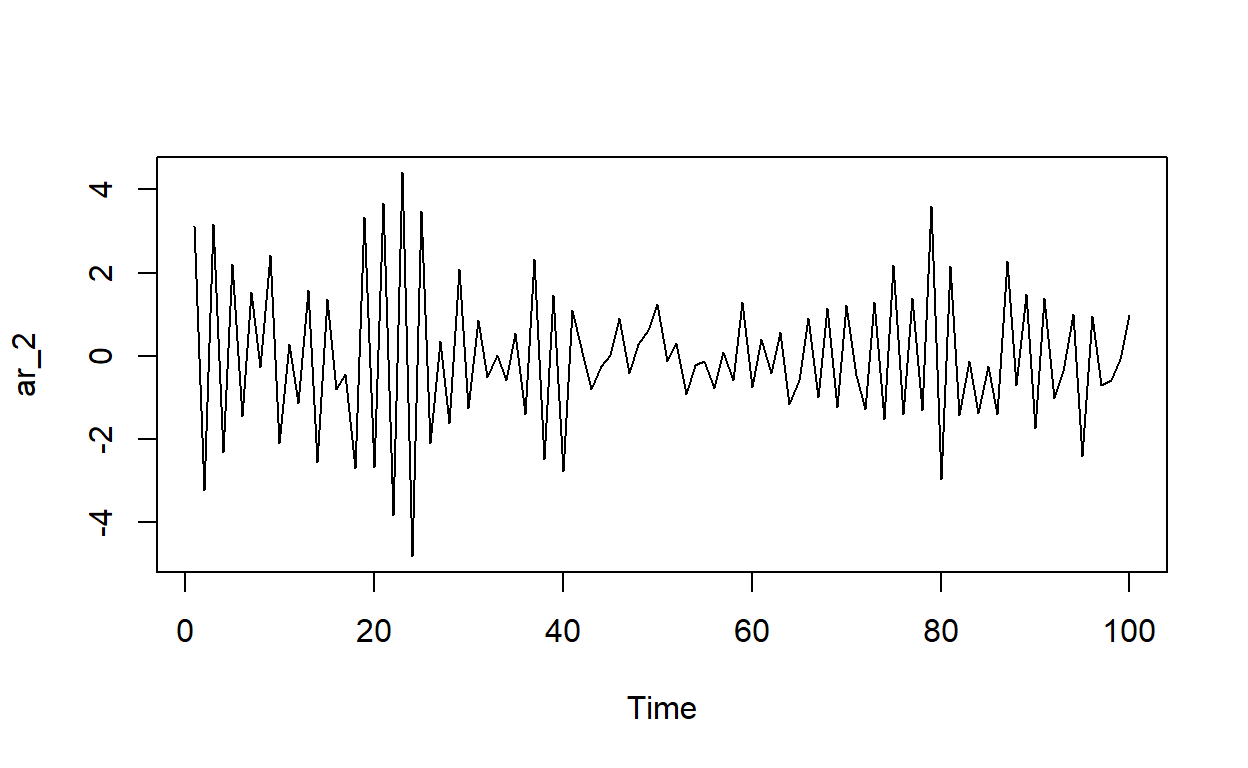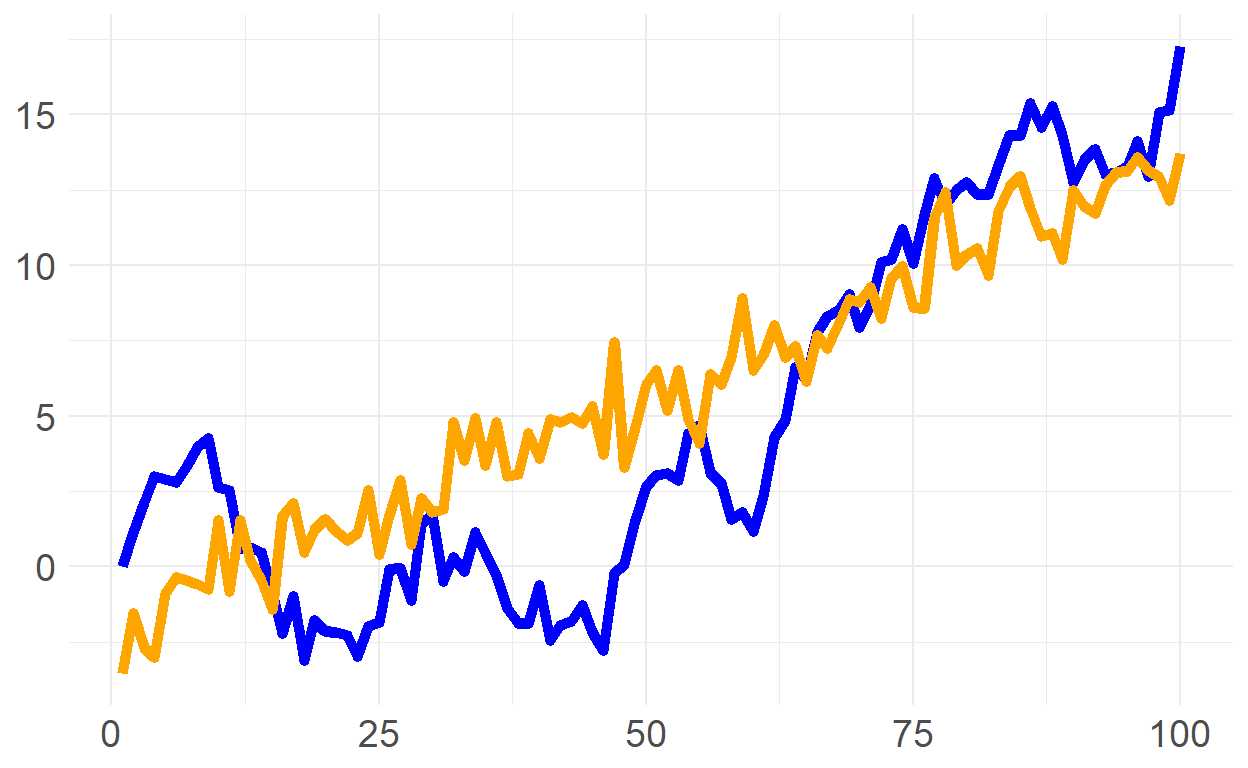# BT PQ P1.T2.21.1 (SET) Non-stationary time series (2021)

Seasonal dummy model, roots of characteristic equation, and transformation (difference versus detrend) of non-stationary process

David Harper https://www.bionicturtle.com/
2022-01-25

### 21.1.1 This is a seasonal model without a trend

21.1.1 The following seasonal dummy model estimates the quarterly growth rate (in percentage terms) of housing starts … The model’s intercept (δ) equals +1.20 and the gamma coefficients are the following: γ(1) = -2.60, γ(2) = +7.90, and γ(3) = +1.80. According to this model, when does the growth rate peak?

``````# similar to GARP's EOC 11.8, there is no trend, only seasonality

c_delta <- 1.20; gamma <- c(-2.6, 7.9, 1.80, 0)
quarters <- rep(1:4, 5)
y = c_delta + gamma[quarters]
ts.plot(y)
``````### 21.1.2 An AR(2) that is stationary as demonstrated by |roots| > 1.0 but also our ability to simulate

21.1.2. Peter wants to model the following AR(2) time series: Y(t) = 0.750Y(t-1) - 0.1250T(t-2) + e(t). He wonders if this AR(2) is stationary. He realizes that he can write this as a log polynomial …

``````# install.packages("polynom", repos = "http://cran.us.r-project.org")
library(polynom)

peters_poly <- polynomial(coef = c(1, -0.75, 0.125))
peters_poly
``````
``1 - 0.75*x + 0.125*x^2 ``
``````solve(peters_poly) # the roots (aka, zeros) are 2 and 4
``````
`` 2 4``
``````ar_2 <-  arima.sim(model=list(order=c(2,0,0),ar = c(-0.75, 0.125)),n = 100)
ts.plot(ar_2)
``````### 21.1.3 Compares a deterministic trend to a stochastic (random walk with drift) trend

21.1.3. Sally considers two series for her model: a linear trend model (aka, deterministic trend), and a random walk with drift. Each is simulated below (n = 100 steps)

``````library(tidyverse)
library(ggthemes)
library(RColorBrewer)

set.seed(28)

n <- 100
x <- 1:n

# white noise
white_noise <- arima.sim(model = list(order = c(0,0,0)), n = 100)

#linear trend
linear_tr <- -1.8 + 0.15*x
time_trend <- linear_tr + white_noise

rw_drift <- arima.sim(model = list(order = c(0,1,0)), n = n-1, mean = 0.4)

trends <- data.frame(
x,
time_trend,
rw_drift
)

p1 <- trends %>% ggplot(aes(x=x)) +
geom_line(aes(y=rw_drift), color = "blue", size = 2) +
geom_line(aes(y=time_trend), color = "orange", size = 2) +
theme_minimal() +
theme(
axis.title = element_blank(),
axis.text = element_text(size = 14)
)

p1
``````